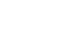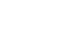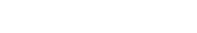## Mathematics Paper 1 Questions and Answers - Bungoma Diocese Mock Exams 2021/2022

INSTRUCTIONS TO CANDIDATES

• The paper contains TWO sections: Section A and B
• Answer ALL questions in section I and STRICTLY ANY FIVE questions from section II.
• All working and answers must be written on the question paper in the spaces provided below each question.
• Show all the steps in your calculations, giving your answers at each stage in the spaces below each question.
• Marks may be awarded for correct working even if the answer is wrong.
• Non-programmable silent electronic calculators and KNEC Mathematical tables may be used except where stated otherwise.

SECTION A (50MKS)
ANSWER ALL THE QUESTIONS IN THIS SECTION

1. Simplify  (1³/₇ − ⁵/₈) + ²/₃ of 1¹/₅     (4mks)
¾ + 1⁵/₇ ÷ ⁴/₇ of 2¹/₃
2. A straight line ax + by = 16 passes through A (2, 5) and B (3, 7). Find the values of a and b (3mks)
3. Simplify     2−x−x     (3mks)
3x2−2x−1
4. Solve for X where 0 ≤ x ≤ = 90°   (2mks)
sin 2x − cos (x−30) = 0
5. Solve for X in                    (3mks)
2x − 4  ≤ 3x + 2 < 10 − x
Hence represent your solution on a number line
6. Two similar cylindrical solids have heights of 18 cm and 24 cm. The volume of the larger cylinder is 320cm3, find the volume of the smaller cylinder (4mks)
7. Solve for X      (3mks)
83x − 2 x 16½x  = ¼
8. A quantity P varies jointly as Q and inversely as on the square root of R. If Q is increased by 10% and R is reduced by 19%, find the percentage change in P (3mks)
9. Okedi sold goods whose marked price is sh. 340,000 at a discount of 2%. He was paid sh. 16660 as commission for the total sales. Calculate the percentage rate of commission (3mks)
10. The interior angle of a regular polygon is three and a half times the exterior angle. Determine the sides of the polygon (3mks)
11. Give that A =, B =;find matrix C where AC = B (3mks)
12. Amoit bought 2 pens and 5 exercise books at a cost of sh. 275. Allan bought 4 such pens and exercise books from the same shop at a cost of sh. 415 by letting sh. X and y to be the costs of a pen and a book respectively, find the cost of each item (4mks )
13. Okech left some money in his will to be shared amongst his wife, son and daughter in the ratio 4:3:2 respectively. If the daughter received sh. 120,000 less than the mother’s share, find the total amount of money Okech left in his will. (2mks)
14. Use tables of reciprocals to find the reciprocal of 0.3758. Hence find the value 3√0.125 correct to 4.S.f (4mks)
0.3758
15. A major sector of a circle subtends an angle of 150 at the centre. The radius of the circle is 7cm and the centre is at O as shownIf the sector is folded into a conical shape, calculate the radius of the cone correct to 1 d.p (3mks)
16. A Kenyan bank buys and sells currencies at the exchange rates below
 Currency Buying (ksh) Selling (ksh) 1 euro 1 us dollar 147.87    74.22 148.00   74.50
An American tourist arrived in Kenya with 24,000 Euros. He converted all the euros to Kenya shillings at the bank. He spent a total sh. 200,000 while in Kenya and converted the rest into US dollars at the bank. Find the amount in dollars that he received. (3mks)

SECTION II (50MKS)
ANSWER ANY FIVE QUESTIONS IN THIS SECTION

1. The diagonals of a rectangle P, Q, R, S intersect at (5, 3). Given that the equation of line PQ is 4y − 9x =13 and that of line PS is y − 4x =5
1. The co-ordinators of P (3mks)
2. The co-ordinates of R (2mks)
3. The equation of line RQ (2mks)
4. The equation of a perpendicular line drawn to meet PR at (5,3) (3mks)
2. A bus left Malaba town at 6.00am and travelled at an average speed of 80km/h towards Nairobi which is 510km away. At 6.30am a salon car left Nairobi the same day following the same route and travelled at average speed of 100km/h towards Malaba. After 1 hour, the car had a puncture which took 15minutes to repair before proceeding with the journey;
Determine
1. The distance covered by the bus in 30minutes (1mks)
2. The time of the day when car met the bus. (6mks)
3. The distance from Nairobi to the point where the car met with the bus (2mks)
4. The time of the day to the nearest minute when the bus got to Nairobi (1mk)
3. Points P, Q and R are a straight line on a level ground. An electricity pole is erected at P with a point X and Y on it. From point X, the angle of depression of point Q is 48° while the angle of depression of R from Y which is 3m above X is 60°
1. Illustrate the position of X, Y, P and R by sketching. (1mk)
2. Hence calculate to 1 d.p.
1. The length XP (3mks)
2. The distance YQ (2mks)
3. The distance PQ (2mks)
4. The angle of elevation of Y from R given that PR = 8cm (2mks)
4.
1. The figure shows a velocity- time graph of a car1. Find the total distance covered by the car in metres (3mks)
2. Calculate the deceleration of the car (3mks)
2. A lorry left kisumu at 8.00am and travelled towards the Nakuru at an average speed of 72km/h. At 8.30am a matatu left kisumu and followed the lorry at an average speed of 96km/h.
Determine the time of the day when the matatu caught up with the lorry (4mks)
5. The table below shows marks scored by 48 students in a geography exam.
 Marks % 30-39 40-49 50-59 60-69 70-79 80-89 Students 6 10 x 9 12 2
1. Determine the value of x (2mks)
2. State the modal class (1mk)
3. Calculate the (3mks)
1. Mean mark
2. Median mark (4mks)
6.
1. Complete the table below for the equation Y = x2 + 3x − 6 where −7 ≤ × ≤ 4
 x −7 −6 −5 −4 −3 −2 −1 0 1 2 3 4 y 4 −6 −2
(3mks)
2. Using the scale 1 cm to represent 1 unit on the X- axis and 1cm to represent 2 units on the Y - axis, draw the graph of y = x2 + 3x − 6 for −7≤ ×≤4 (4mks)
3. Use your graph to solve for x in
x2 + 3x − 6 =0 (2mks)
4. State the;
1. Turning point of the curved (1mk)
2. Equation of the line symmetry (1mk)
7. the figure shows triangle ABC inscribed in a circle where AC = 10cm, BC = 7cm and AB =11cmCalculate correct 1 d p ( use π = 22/7 )
1. The size of the angle CAB (4mks)
2. The radius of the circle (2mks)
3. Hence, find the area of the shaded region (4mks)
8. ABCDEFGA is a belt tied around two wheels whose centres are O and Q forming a pulley system. Given that Q =36cm, AO = 5cm BQ = 7cm. calculate correct 1 d.p (Take π = 22/7 )1. Angle AOQ (3mks)
2. The length of the belt in contact with
1. The wheel whose centre is O (2mks)
2. The wheel whose centre is Q (2mks)
3. The length of AB, hence the total length of the belt (3mks)## MARKING SCHEME

1. Num (¹⁰/₇ − ⁵/₈) + ²/₃ of ⁶/₅
= 80 − 35 + 4
56       5
45/564/5
= 225 + 224
280
449
280
Den  ¾ + ¹²/₇ ÷ ⁴/₇ of ⁷/₃
= ¾ + ¹²/₇ × ⁷/₄ of ¾
= ¾ + ¹²/₇ × ¾
= 21 + 36
28
57/28
449/280 × 28/57449/570
2. 2a + 5b = 16
3a + 7b = 16
6a + 15b = 48
6a + 14b = 32
b = 16
a = −32
3. 2  − x  − x2 = 2  − 2x + x  − x2
= 2(1  −  x) + x ( 1  − x)
= (2 + x)(1 − x)
3x2  − 3x + x  − 1
= 3x(x  − 1) +1(x  − 1)
=(3x+1)(x −1)
∴  =(2 + x) (1 − x2)
(3x + 1)(x  − 1)
=  − 2 + x
3x + 1
4. 2x + x − 30 = 90
3x = 120
x = 40
5. 2x − 4 ≤ 3x + 2
−6 ≤ x
3x + 2 < 10 − x
4x < 8
x < 26. LSF = 18/24 = ¾
VSF = (¾)327/64
27/64x/320
x = 135cm3
7.  23(3x − 2) x 24(½x)  = 4−1
23(3x − 2) x 24(½x)  = 2−2
9x − 6 + 2x = −2
11x = 4
x = 4/11
8.  P ∝
√R
P = KQ
√R
New P =    1.1 P
√0.81
= 1.1 P = 1.2222P
0.9
%Increase = 0.2222 × 100%
= 22.22%
9.  Sales = 100 − 2 × sh 340,000
100
= 98/100 × sh 340,000
= Sh 333,200
%Commission =  16660  × 100%
333200
=5%
10. Let ext angle = x
3½x + x = 180°
4.5x = 180
x = 40°
11. AC = B
C = A−1 = B12. 2x + 5y = 275
4x + 7y = 415
8x + 20y = 1100
8x + 14y = 830
6y = 270
y = 45
x = 25
13. W : S : D
4 : 3 : 2
Let total amount = x
(4/9 − 2/9)x = sh 120,000
2/9x = 120,000
x = Sh 540,000
14. 0.3758 =    1
0.3758
=          1
1 × 3.758 × 10−1
= 1 × 0.2661 × 101
= 2.661
3√0.125 = 0.05
∴ = 2.661 ×  0.5
= 1.3305
= 1.331
15.  Area = 150/360 ×  22/7 ×  7 ×  7
= 64..17cm2
∴ 64.17 = 22/7 × R × 7
2.917 = R
2.9 = R
16.  Amount on arrival = 24000  × 147.87
= Sh. 3,548,880
Balance = Sh. 3,548,880 − Sh 200,000
= Sh 3,348,880
74.50
= 44951dollars
17.1. y − 4x = 5 ......(i)
4y − 9x = 13 .....(ii)
4y − 16x = 20
− 4y − 9x = 13
−7x = 7
x = −1
y = 1
Hence P (−1,1)
2. x = 2(5) − (−1) = 11
y = 2(3) −1      = 5
Hence R(11,5)
3. SP // RQ
Hence M1 = M2 = 4
∴ 4 = y − 11
x − 5
y − 11 = 4(x−5)
y = 4x − 20 + 11
y = 4x − 9

4. PR ∴ P(−1,1) and (5,3)
Grad = M1 = 3−1 = 2 = 1
5+1    5    3
M2  = −3
Hence −3 = y − 3
x −5
y−3 = −3(x − 5)
y = −3x + 15 + 3
y = − 3x + 18
18.1. Distance covered = 80 × ½h             ½h + 1h + ¼h = 1¾h
= 40km
2. Relative distance = 510 − (80 × 1¾ + 100 × 1)
= 510 − (80 × 7/4 + 100)
= 510 − (140 + 100)
= 510 − 240
= 270km
Time taken =    270km           = 270
(100 + 80)km/h    180
= 1½hrs
Time of day = 6.00am + ½hr + 1hr + ¼hr + 1½hr
= 6.00am + 3hr 15mins
= 9.15a.m
3. Distance from NRB = 100 × 1 + 100 × 3/2
= 100 + 150
= 250km
4.  Time taken = 510km  = 6.375hr
80km/h
= 6hr 23mins
Time of day = 6.00am + 6hrs 23 mins
= 12.23pm
19.

1.2.
1. Tan 42 = h/t
h1 = t tan 42°
Tan 30 =   h
t + 3
h2 = tan 30(t+3)
Hence h1 = h2
t tan 42 = tan 30(t+3)
0.9004t = 0.5774(t+3)
0.9004t = 0.5774t + 1.7322
0.9004t − 0.5774t = 1.7322
0.323t = 1.7322
t = 1.7322
0.323
= 5.363
≅ 5.4m
2. Cos 30° = 8.362
YR
YR = 8.362
cos 30
= 9.656 = 9.7m
3. PQ = (3 + 5.362) tan 30°
= 8.362 tan 30
= 4.828 = 4.8m
4. Tan x = 8.362
8
x = tan−1 (8.362)
8
= 46.27°
= 46.3°
20.
21.
22.
1.
 x −7 −6 −5 −4 −3 −2 −1 0 1 2 3 4 y 22 12 4 −2 −6 −8 −8 −6 −2 4 12 22

2.23.
1. Area of √14(14−11)(14−10)(14−7)                     Alternative method
Δ ABC = √(14 × 3 × 4 ×7                                  Cos A = 11² + 10² − 7²
=√1156                                                               2 × 11 × 10
= 34.292                                                           = 172/220 = 0.7818
A = ½ab sin θ                                                            A = Cos−1 (0.7818)
34.292 = ½ × 11 × 10 sin A                                          = 38.57
Sin A = 34.292 × 2                                                    = 38.6°
110
= 0.6235
A = sin−1 0.6235
= 38.57
= 38.6°
2 sin A
=         7
2 sin 38.57
= 5.614
= 5.6
3. Shaded Area = πr2 − ½ab sinθ                              Area of circle and evidence of subtraction
= 22/7 × 5.614² − 34.292
= 99.05 − 34.292
= 64.758
= 64.8
24.Sin x = 2/36 = 0.05556
x = sin−1 (2/36)
= 3.185°
1. < AOQ = 90 + 3.185
= 93.185 = 93.2
<AOE = 360 − 2 × 93.185
= 360 − 186.37
= 173.63   ≅ 173.6
2.
1. Arc length = θ/360 × 2πr
= 173.63 × 2 × 22 × 5
360              7
= 15.16      ≅ 15.2
2. Arc length =  186.37 × 2 × 22 × 5
360              7
= 22.78   ≅ 22.8
3. AB = √(362 − 22)
= √1292
= 35.94    ≅ 35.9
Total length = 15.16 + 22.78 + 2 × 35.94
= 109.82

• ✔ To read offline at any time.
• ✔ To Print at your convenience
• ✔ Share Easily with Friends / Students